Courses

# Solutions of Refraction of Light (Page No- 262 & 263) - Physics By Lakhmir Singh, Class 10 Class 10 Notes | EduRev

## Class 10 : Solutions of Refraction of Light (Page No- 262 & 263) - Physics By Lakhmir Singh, Class 10 Class 10 Notes | EduRev

The document Solutions of Refraction of Light (Page No- 262 & 263) - Physics By Lakhmir Singh, Class 10 Class 10 Notes | EduRev is a part of the Class 10 Course Class 10 Physics Solutions By Lakhmir Singh & Manjit Kaur.
All you need of Class 10 at this link: Class 10

Lakhmir Singh Physics Class 10 Solutions Page No:262

Question 20: A lens has a focal length of, -10 cm. What is the power of the lens and what is its nature ?

Solution : f = -10 cm = -0.1 m
P = 1/f = 1/(-0.1) = -10 D
It is a concave lens.

Question 21: The focal length of a lens is +150 mm. What kind of lens is it and what is its power ?

Solution : f = +150 mm = +0.15 m
It is a convex lens since its focal length is positive.
P = 1/f = 1/0.15 = +6.66 D

Question 22: Fill in the following blanks with suitable words :
(a) The reciprocal of the focal length in metres gives you the……….. of the lens, which is measured in
(b) For converging lenses, the power is……… while for diverging lenses, the power is………….

Solution : (a) power, dioptres.
(b) positive, negative.

Question 23: An object of height 4 cm is placed at a distance of 15 cm in front of a concave lens of power, -10 dioptres. Find the size of the image.

Solution :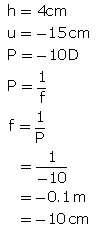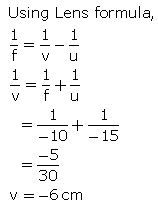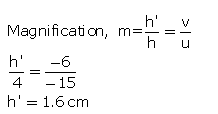Question 24: An object of height 4.25 mm is placed at a distance of 10 cm from a convex lens of power +5 D. Find (i) focal length of the lens, and (ii) size of the image.

Solution :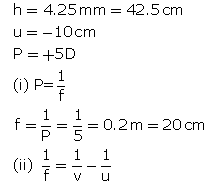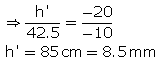Question 25: A convex lens of power 5 D and a concave lens of power 7.5 D are placed in contact with each other. What is the :
(a) power of this combination of lenses ?
(b) focal length of this combination of lenses ?

Solution :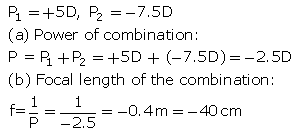Question 26: A convex lens of focal length 25 cm and a concave lens of focal length 10 cm are placed in close contact with one another.
(a) What is the power of this combination ?
(b) What is the focal length of this combination ?
(c) Is this combination converging or diverging ?

Solution :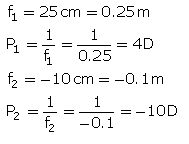(a) Power of the combination: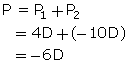(b) Focal length of the combination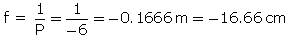(c) The combination has negative focal length, so it is diverging.

Question 27: The power of a combination of two lenses X and Y is 5 D. If the focal length of lens X be 15 cm :
(a) calculate the focal length of lens Y.
(b) state the nature of lens Y.

Solution :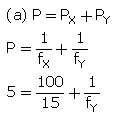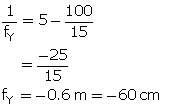(b) Lens Y is a concave lens since it has negative focal length.

Question 28: Two lenses A and B have focal lengths of + 20 cm and, -10 cm, respectively.
(a) What is the nature of lens A and lens B ?
(b) What is the power of lens A and lens B ?
(c) What is the power of combination if lenses A and B are held close together ?

Solution :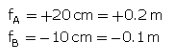(a) Lens A is a convex lens (positive focal length) and lens B is a concave lens (negative focal length).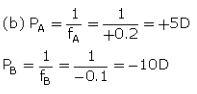(c) Power of combination
P = PA +PB = + 5D + (-10D) = -5D

Question 29: (a) What do you understand by the power of a lens ? Name one factor on which the power of a lens depends.
(b) What is the unit of power of a lens ? Define the unit of power of a lens.
(c) A combination of lenses for a camera contains two converging lenses of focal lengths 20 cm and 40 cm and a diverging lens of focal length 50 cm. Find the power and focal length of the combination.

Solution : (a) Power of a lens is a measure of the degree of convergence or divergence of light rays falling in it.

Power of a lens depends on its focal length.

(b) Unit of power of a lens is dioptre.

One dioptre is the power of a lens whose focal length is 1 metre.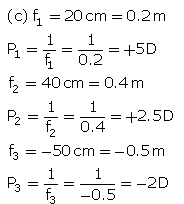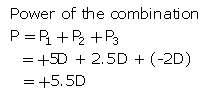Focal length orthr combination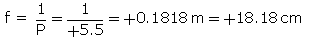Question 30: (a) Two lenses A and B have power of (i) + 2 D and (ii) – 4 D respectively. What is the nature and focal length of each lens ?
(b) An object is placed at a distance of 100 cm from each of the above lenses A and Calculate (i) image distance, and (ii) magnification, in each of the two cases.

Solution :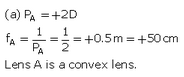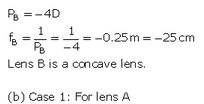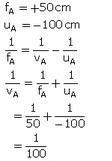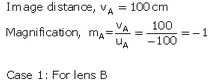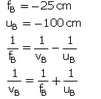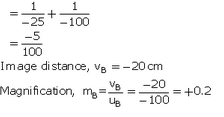Lakhmir Singh Physics Class 10 Solutions Page No:263

Question 39: The optical prescription for a pair of spectacles is :
Right eye : – 3.50 D Left eye : – 4.00 D
(a) Are these lenses thinner at the middle or at the edges ?
(b) Which lens has a greater focal length ?
(c) Which is the weaker eye ?

Solution : (a) These lenses have negative powers and hence negative focal lengths, so they are concave lenses.
Concave lenses are thinner in the middle.
(b) Lens of lower power has greater focal length.
So, -3.50 D lens has greater focal length.
(c) Left eye is the weaker one because it needs a lens of greater power for its correction.

Question 40: A person got his eyes tested by an optician. The prescription for the spectacle lenses to be made reads :
Left eye : + 2.50 D Right eye : + 2.00 D
(a) State whether these lenses are thicker in the middle or at the edges.
(b) Which lens bends the light rays more strongly ?
(c) State whether these spectacle lenses will converge light rays or diverge light rays.

Solution : (a) These lenses have positive powers and hence positive focal lengths, so they are convex lenses.
Convex lenses are thicker in the middle.
(b) Lens of greater power bends light rays more quickly.
So, +2.50 D lens bends light rays more quickly.
(c) These spectacle lenses will converge the light rays because these are convex lenses.

Offer running on EduRev: Apply code STAYHOME200 to get INR 200 off on our premium plan EduRev Infinity!

94 docs

,

,

,

,

,

,

,

,

,

,

,

,

,

,

,

,

,

,

,

,

,

,

,

,

;